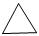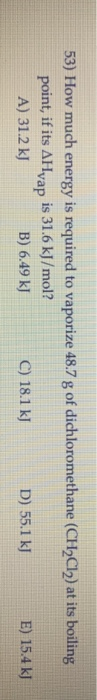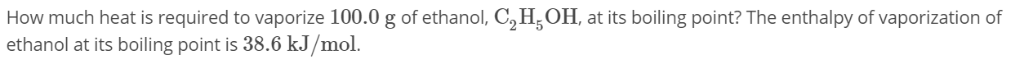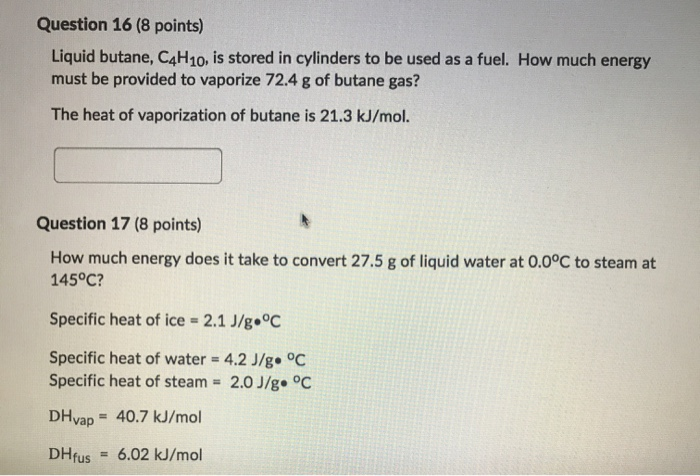# How much energy is required to vaporize 158 g of butane (C4H10) at its boiling point,...

How much energy is required to vaporize 158 g of butane (C4H10) at its boiling point, if its ΔHvap is 24.3 kJ/mol

Molar mass of C4H10,
MM = 4*MM(C) + 10*MM(H)
= 4*12.01 + 10*1.008
= 58.12 g/mol

mass(C4H10)= 158 g

use:
number of mol of C4H10,
n = mass of C4H10/molar mass of C4H10
=(158.0 g)/(58.12 g/mol)
= 2.719 mol

use:
Q = n*Hvap
= 2.719 mol * 24.3 KJ/mol
= 66.1 KJ

#### Earn Coin

Coins can be redeemed for fabulous gifts.

Similar Homework Help Questions
• ### How much energy is required tp vaporize 48.7 g pf dichloromethane at its boiling point, if...How much energy is required tp vaporize 48.7 g pf dichloromethane at its boiling point, if tis Hvap is 31.6 kJ/mol?

• ### 53) How much energy is required to vaporize 48.7 g of dichloromethane (CH2Cl2) at its boiling...53) How much energy is required to vaporize 48.7 g of dichloromethane (CH2Cl2) at its boiling point, if its AHvap is 31.6 kJ/mol? A) 31.2 kJ B) 6.49 kJ C) 18.1 kJ D) 55.1 kJ E) 15.4 kJ

• ### How much heat is required to vaporize 100.0 g of ethanol, C2H,OH, at its boiling point?...How much heat is required to vaporize 100.0 g of ethanol, C2H,OH, at its boiling point? The enthalpy of vaporization of ethanol at its boiling point is 38.6 kJ/mol.

• ### Butane (C4H10) has a heat of vaporization of 22.44 kJ/mol and a normal boiling point of -0.4 C

Butane (C4H10) has a heat of vaporization of 22.44 kJ/mol and a normal boiling point of -0.4 C. A 250 mL sealed flask contains 0.5 g of butane at -22 C. How much butane is present as a liquid? If the butane is warmed to 25 C, how much is present as a liquid?

• ### Butane (C4H10) has a heat of vaporization of 22.44 kJ/mol and a normal boiling point of...

Butane (C4H10) has a heat of vaporization of 22.44 kJ/mol and a normal boiling point of -0.4 ∘C. A 250 mL sealed flask contains 0.7 g of butane at −22∘C. How much butane is present as a liquid at −22∘C? Express your answer using two significant figures. If the butane is warmed to 25 ∘C, how much liquid butane is present?

• ### How much heat (in kJ) is required to raise the temperature of 202.8 g of ethanol...

How much heat (in kJ) is required to raise the temperature of 202.8 g of ethanol (mw=46.07) from 40.34 °C to its boiling point of  78.37 °C and then vaporize it completely creating ethanol gas at the boiling temperature? (specific heat = 2.46 J/g°C, ΔHvap = 48.6 kJ/mol)

• ### How much heat (in kJ) is required to raise the temperature of 288.7 g of ethanol...

How much heat (in kJ) is required to raise the temperature of 288.7 g of ethanol (mw=46.07) from 39.38 °C to its boiling point of 78.37 °C and then vaporize it completely creating ethanol gas at the boiling temperature? (specific heat = 2.46 J/g°C, ΔHvap = 48.6 kJ/mol)

• ### How much heat (in kJ) is required to raise the temperature of 124.9 g of ethanol...

How much heat (in kJ) is required to raise the temperature of 124.9 g of ethanol (mw=46.07) from 30.24 °C to its boiling point of  78.37 °C and then vaporize it completely creating ethanol gas at the boiling temperature? (specific heat = 2.46 J/g°C, ΔHvap = 48.6 kJ/mol)

• ### How much heat is required to vaporize 2.00 L of CCl4 at its normal boiling point?

The following data are given for . Normal melting point, -23C , normal boiling point, 77C ; density of liquid 1.59g/ml ;vapor pressure at 25C , 110 Torr .How much heat is required to vaporize 2.00 L of CCl4 at its normal boiling point?Answer in kJ

• ### someone hlep pelase Question 16 (8 points) Liquid butane, C4H10, is stored in cylinders to be...someone hlep pelase Question 16 (8 points) Liquid butane, C4H10, is stored in cylinders to be used as a fuel. How much energy must be provided to vaporize 72.4 g of butane gas? The heat of vaporization of butane is 21.3 kJ/mol. Question 17 (8 points) How much energy does it take to convert 27.5 g of liquid water at 0.0°C to steam at 145°C? Specific heat of ice = 2.1 J/g °C Specific heat of water = 4.2 J/g....

Free Homework App